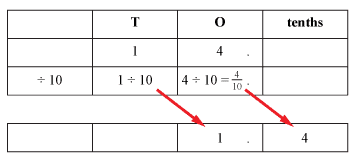#### Dividing whole numbers by 10, 100 or 1000

When 14 is divided by 10, the 1 in the tens place is divided by 10, and the 4 in the ones place is divided by 10.

10 ÷ 10 = 1 and 4 ÷ 10 = $$\dfrac{4}{10}$$ or 0.4.Detailed description

14 ÷ 10 = 1.4